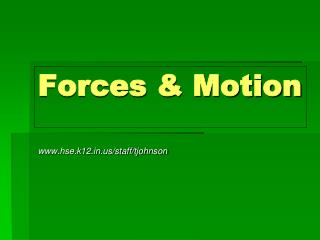DownloadDownload PresentationForces & Motion

# Forces & Motion

Download Presentation## Forces & Motion

- - - - - - - - - - - - - - - - - - - - - - - - - - - E N D - - - - - - - - - - - - - - - - - - - - - - - - - - -
##### Presentation Transcript

1. Forces & Motion www.hse.k12.in.us/staff/tjohnson

2. Newton’s First Law • Newton’s First Law of Motion • An object at rest will remain at rest and an object in motion will continue moving at a constant velocity unless acted upon by a net force.

3. Newton’s Second Law • Newton’s Second Law of Motion • The acceleration of an object is directly proportional to the net force acting on it and inversely proportional to its mass. F = ma

4. F a m Newton’s Second Law F m F = ma F: force (N) m: mass (kg) a: accel (m/s2) 1 N = 1 kg ·m/s2

5. F a m Calculations • What force would be required to accelerate a 40 kg mass by 4 m/s2? GIVEN: F = ? m = 40 kg a = 4 m/s2 WORK: F = ma F = (40 kg)(4 m/s2) F = 160 N

6. F a m Calculations • A 4.0 kg shotput is thrown with 30 N of force. What is its acceleration? GIVEN: m = 4.0 kg F = 30 N a = ? WORK: a = F ÷ m a = (30 N) ÷ (4.0 kg) a = 7.5 m/s2

7. F a m Calculations • Mr. Keller weighs 745 N. What is his mass? GIVEN: F(W) = 745 N m = ? a(g) = 9.8 m/s2 WORK: m = F ÷ a m = (745 N) ÷ (9.8 m/s2) m = 76.0 kg